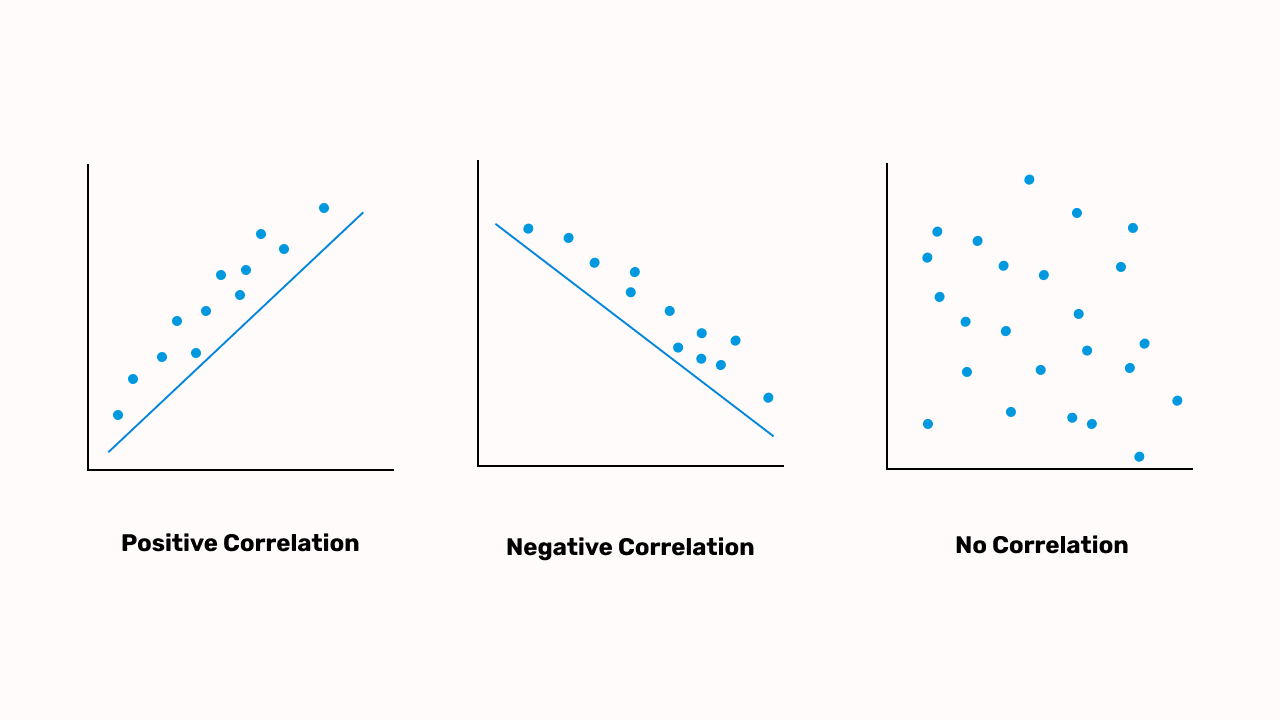UIUX 360 / 15-September-2022 / minute read

# Correlation

Correlation is a statistical measurement and relationship between two sets of data or two variables. A correlation can be positive (as one variable or the other increases) or negative (as one variable or the other decreases).## What is Correlation?

### Overview

Correlation is a statistical measurement and relationship between two sets of data or two variables. A correlation can be positive (as one variable or the other increases) or negative (as one variable or the other decreases). Correlation does not imply that the motion of one variable causes the other to move.

In the finance industry, correlation is a measurement of the degree to which two securities move in relation to each other. Correlations are used in advanced portfolio management, which is calculated as the correlation coefficient, which should have a value between -1.0 and +1.0. There are three types of correlation: positive and negative correlation, Linear and non-linear correlations, and Simple/Multiple/Partial Correlation.

Positive Correlation - Two random variables come closer together. There is a positive relationship between height and weight: weight increases as height increases.

Negative Correlation - As one random variable increases, the other decreases. There is a negative correlation between speed and the time taken to reach somewhere; as the speed increases, it takes less time to reach the destination.

No correlation - There is no linear relationship between two random variables. There is no correlation between No. of cities in Canada and the number of fish in the ocean.### Need help developing a similar project?

Book a call with us and get the party started!